### Practice Set 4.2 Geometric Constructions Class 10th Mathematics Part 2 MHB Solution

Practice Set 4.2

1. Construct a tangent to a circle with centre P and radius 3.2 cm at any point M on it.…
2. Draw a circle of radius 2.7 cm. Draw a tangent to the circle at any point on it.…
3. Draw a circle of radius 3.6 cm. Draw a tangent to the circle at any point on it without…
4. Draw a circle of radius 3.3 cm Draw a chord PQ of length 6.6 cm. Draw tangents to the…
5. Draw a circle with centre P and radius 3.4 cm. Take point Q at a distance 5.5 cm from…
6. Draw a circle with radius 4.1 cm. Construct tangents to the circle from a point at a…

###### Practice Set 4.2

Question 1.

Construct a tangent to a circle with centre P and radius 3.2 cm at any point M on it.

Steps of construction:

i. Draw a circle with center P and radius 3.2 cm

ii. Take a point M on the circle, Join PM.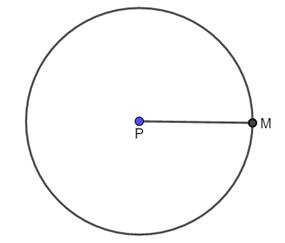iii. Draw AB ⊥ PM such that AB passes through M, AB is required tangent.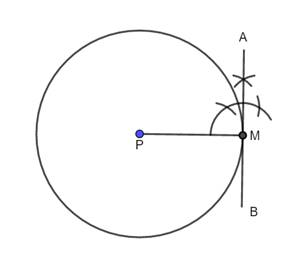Question 2.

Draw a circle of radius 2.7 cm. Draw a tangent to the circle at any point on it.

Steps of construction:

i. Draw a circle with center P and radius 2.7 cm

ii. Take a point M on the circle, Join PM.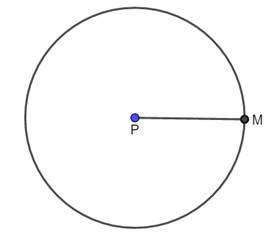iii. Draw AB ⊥ PM such that AB passes through M, AB is required tangent.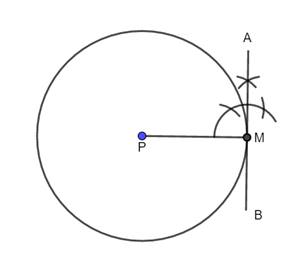Question 3.

Draw a circle of radius 3.6 cm. Draw a tangent to the circle at any point on it without using the centre.

i. Draw a circle of radius 3.6 cm. Take any point C on it.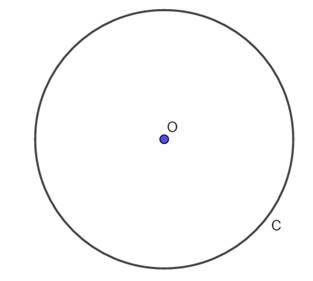ii. Draw chord CB and an inscribed ∠CAB .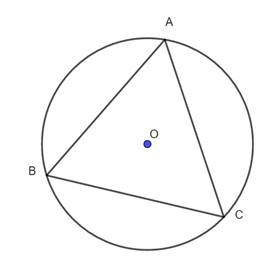iii. With the centre A and any convenient radius draw an arc intersecting the sides of ∠BAC in points M and N.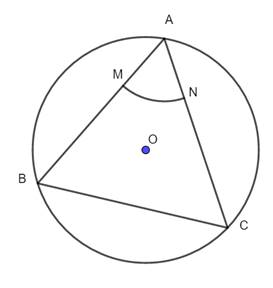iv. Using the same radius and centre C, draw an arc intersecting the chord CB at point R.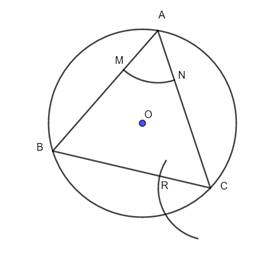v. Taking the radius equal to d(MN) and centre R, draw an arc intersecting the arc drawn in the previous step. Let D be the point of intersection of these arcs. Draw line CD. Line CD is the required tangent to the circle.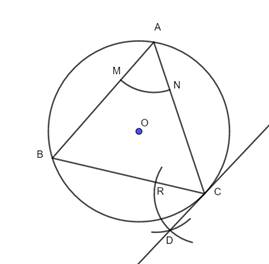Question 4.

Draw a circle of radius 3.3 cm Draw a chord PQ of length 6.6 cm. Draw tangents to the circle at points P and Q. Write your observation about the tangents.

Here chord = 6.6 = 2 × 3.3 cm = 2 × radius, hence PQ is diameter of the circle.

Steps of construction:

i. Draw a circle with center O, and radius 3.3 cm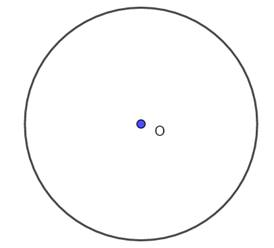ii. Draw a diameter PQ passing through center.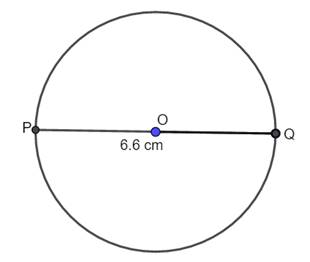iii. Draw AB ⊥ OQ and CD ⊥ OP , such that AB and CD pass through Q and P respectively.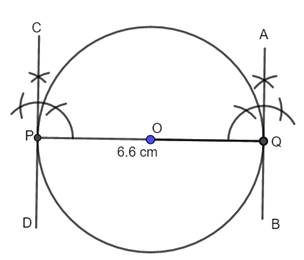iv. AB and CD are required tangents.

Observation: AB || CD, i.e. tangents at opposite ends of diameter are parallel.

Question 5.

Draw a circle with centre P and radius 3.4 cm. Take point Q at a distance 5.5 cm from the centre. Construct tangents to the circle from point Q.

Steps of construction:

i. Draw a circle with center P and radius 3.4 cm.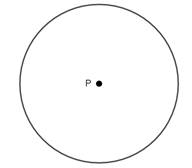ii. Take a point Q outside the circle such that PQ = 5.5 cm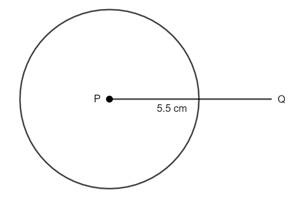iii. Draw the perpendicular bisector of PQ, which bisects PQ at O.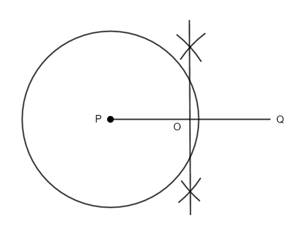iv. Taking O as center and OP = OQ as radius, draw another circle, which intersects the previous circle at A and B.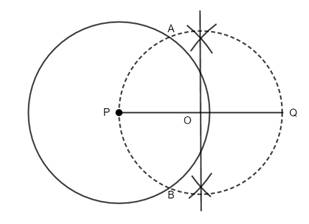v. Join AQ and BQ, which are required tangents.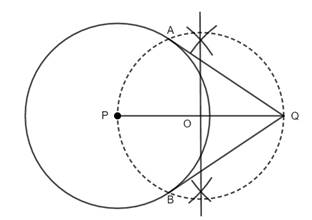Question 6.

Draw a circle with radius 4.1 cm. Construct tangents to the circle from a point at a distance 7.3 cm from the centre.

Steps of construction:

i. Draw a circle with center P and radius 4.1 cm.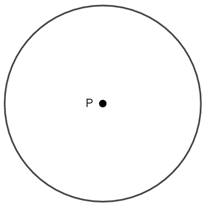ii. Take a point Q outside the circle such that PQ = 7.3 cm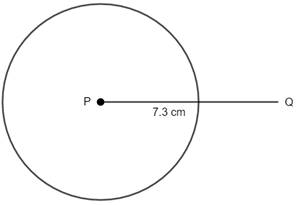iii. Draw the perpendicular bisector of PQ, which bisects PQ at O.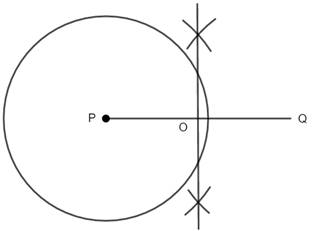iv. Taking O as center and OP = OQ as radius, draw another circle, which intersects the previous circle at A and B.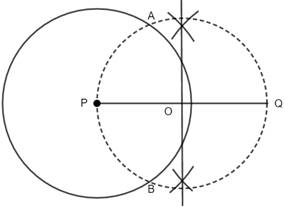v. Join AQ and BQ, which are required tangents.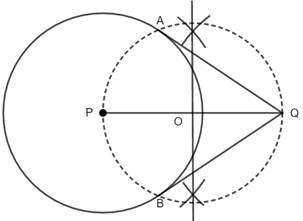## PDF FILE TO YOUR EMAIL IMMEDIATELY PURCHASE NOTES & PAPER SOLUTION. @ Rs. 50/- each (GST extra)

SUBJECTS

HINDI ENTIRE PAPER SOLUTION

MARATHI PAPER SOLUTION
SSC MATHS I PAPER SOLUTION
SSC MATHS II PAPER SOLUTION
SSC SCIENCE I PAPER SOLUTION
SSC SCIENCE II PAPER SOLUTION
SSC ENGLISH PAPER SOLUTION
SSC & HSC ENGLISH WRITING SKILL
HSC ACCOUNTS NOTES
HSC OCM NOTES
HSC ECONOMICS NOTES
HSC SECRETARIAL PRACTICE NOTES

2019 Board Paper Solution

HSC ENGLISH SET A 2019 21st February, 2019

HSC ENGLISH SET B 2019 21st February, 2019

HSC ENGLISH SET C 2019 21st February, 2019

HSC ENGLISH SET D 2019 21st February, 2019

SECRETARIAL PRACTICE (S.P) 2019 25th February, 2019

HSC XII PHYSICS 2019 25th February, 2019

CHEMISTRY XII HSC SOLUTION 27th, February, 2019

OCM PAPER SOLUTION 2019 27th, February, 2019

HSC MATHS PAPER SOLUTION COMMERCE, 2nd March, 2019

HSC MATHS PAPER SOLUTION SCIENCE 2nd, March, 2019

SSC ENGLISH STD 10 5TH MARCH, 2019.

HSC XII ACCOUNTS 2019 6th March, 2019

HSC XII BIOLOGY 2019 6TH March, 2019

HSC XII ECONOMICS 9Th March 2019

SSC Maths I March 2019 Solution 10th Standard11th, March, 2019

SSC MATHS II MARCH 2019 SOLUTION 10TH STD.13th March, 2019

SSC SCIENCE I MARCH 2019 SOLUTION 10TH STD. 15th March, 2019.

SSC SCIENCE II MARCH 2019 SOLUTION 10TH STD. 18th March, 2019.

SSC SOCIAL SCIENCE I MARCH 2019 SOLUTION20th March, 2019

SSC SOCIAL SCIENCE II MARCH 2019 SOLUTION, 22nd March, 2019

XII CBSE - BOARD - MARCH - 2019 ENGLISH - QP + SOLUTIONS, 2nd March, 2019

HSC Maharashtra Board Papers 2020

(Std 12th English Medium)

HSC ECONOMICS MARCH 2020

HSC OCM MARCH 2020

HSC ACCOUNTS MARCH 2020

HSC S.P. MARCH 2020

HSC ENGLISH MARCH 2020

HSC HINDI MARCH 2020

HSC MARATHI MARCH 2020

HSC MATHS MARCH 2020

SSC Maharashtra Board Papers 2020

(Std 10th English Medium)

English MARCH 2020

HindI MARCH 2020

Hindi (Composite) MARCH 2020

Marathi MARCH 2020

Mathematics (Paper 1) MARCH 2020

Mathematics (Paper 2) MARCH 2020

Sanskrit MARCH 2020

Important-formula

THANKS